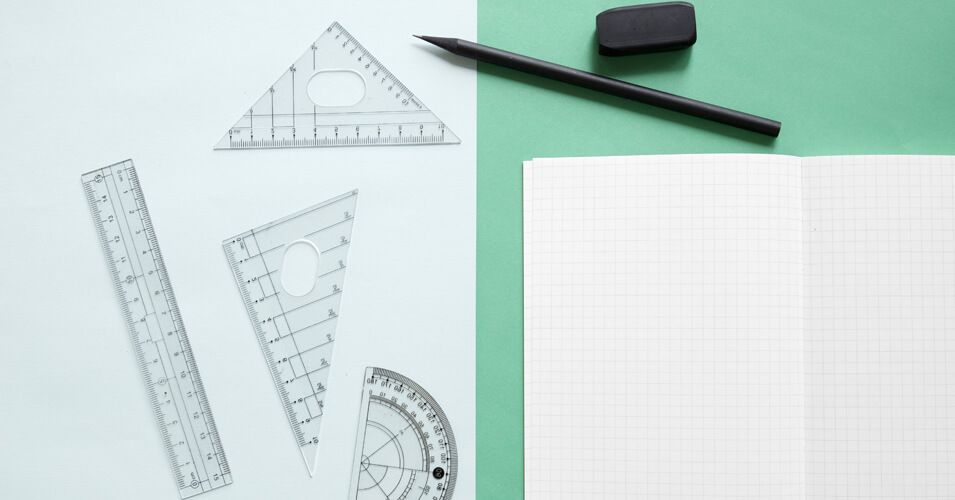• Save

# Coordinate Geometry Questions and AnswersIn competitive exams like SSC, IAS, RAS, UPSC, RRB, etc are asked in Coordinate Geometry questions, which is a core part of the Quantitative Aptitude section, it tests a candidate on concepts in a comprehensive way - geometric shapes. Graphical representation, the distance between digits, section formula, intercept, circle, etc. Generally, if you are expecting to score almost all the marks in the Coordinate Geometry questions, then you must first understand the complexity of these questions. Here you can practice by solving the coordinated geometry questions with the help of the following blogs.

Here you can also practice with other Mock Tests through Test Series.

## Important Coordinate Geometry Questions

Q :

Find k, if the line 2x - 3y = 11 is perpendicular to the line 3x + ky = -4?

(A) -1

(B) 2

(C) -2

(D) 1

Q :

What is the reflection of the point (-3, 1) in the line x = -2?

(A) (1, 1)

(B) (-3 , 5)

(C) (-1, 1)

(D) ( -3 , -5)

Q :

The graphs of x = a and y = b intersect at

(A) (-a, b)

(B) (b, a)

(C) ( a, b )

(D) (a, -b)

Q :

What is the equation of line whose slope is -1/2 and passes through the intersection of the lines x-y = -1 and 3x-2y = 0?

(A) x + 2y = -8

(B) 3x + y = -7

(C) x + 2y = 8

(D) 3x + y = 7

Q :

A line passing through the origin perpendicularly cuts the line 3x – 2y = 6 at point M. Find the co-ordinates of M.

(A) $$\left({-18\over13},{-12\over13} \right)$$

(B) $$\left({-18\over13},{12\over13} \right)$$

(C) $$\left({18\over13},{12\over13} \right)$$

(D) $$\left({18\over13},{-12\over13} \right)$$

Q :

The linear equation such that each point on its graph has an ordinate four times its abscissa is:

(A) x = 4y

(B) x + 4y = 0

(C) y + 4x = 0

(D) y = 4x

Q :

The point P(a, b) is first reflected in origin to P1 and P1 is reflected in Y-axis to (4, -3). What are the coordinates of point P?

(A) ( -4 , 3 )

(B) ( 3 , 4 )

(C) ( 4 , 3 )

(D) ( -3 , 4 )

Q :

What is the slope of the line perpendicular to the line passing through the points (-5, 1) and (-2, 0)?

(A) 3

(B) -1/3

(C) -3

(D) 1/3

Q :

If (2x)(2y) = 8 and (9x)(3y) = 81 , then ( x , y ) is :

(A) ( 1, 1 )

(B) ( 2, 2 )

(C) ( 1, 2 )

(D) ( 2, 1 )

Q :

What is the equation of a line having slope -1/3 and y-intercept equal to 6?

(A) x - 3y = 6

(B) x + 3 y = -18

(C) x + 3 y = 18

(D) x - 3y = -6